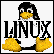### .. binary to hex ..

There are no hexadecimal numbers to be found anywhere inside the computer. Internally everything is in binary numbers. Hex is used to "represent" the binary numbers which are being used. It is a shorthand only, but it is in universal use among programmers, and if you need to get close to a computer you should at least know how it is used. If you are writing color values for web pages, you will certainly need to use hex notation, although you will never need to do things like add hex numbers.

Hexadecimal numbers have values from zero through F, as follows..

0 1 2 3 4 5 6 7 8 9 A B C D E F

.. with equivalent decimal values of..

0 1 2 3 4 5 6 7 8 9 10 11 12 13 14 15

.. the equivalent 4-bit binary numbers are..

0000 0001 0010 0011 0100 0101 0110 0111 1000 1001 1010 1011 1100 1101 1110 1111

### .. CPU register ..

Typically a 16-bit CPU register (there are a dozen registers in use in a CPU) might contain the following number...

0 0 0 0 1 1 1 0 1 1 1 0 1 0 1 1

If you must know, the above binary number is "3819" in decimal. You can figure that out by adding the values of each column which has a "1" - the decimal value 3819 results from

2048 + 1024 + 512 + 0 + 128 + 64 + 32 + 0 + 8 + 0 + 2 + 1 = 3819

Since it is almost impossible to make sense out of long strings of binary numbers, like the 000111011101011-bin above, programmers divide binary numbers up into groups of four, like this ...

0000, 1110, 1110, 1011

.. and then represent each of the groups of four as a base-16 (hexadecimal) number, since the highest value of a 4-bit binary number is 15-decimal. Now this is somewhat easier to read.

0,E,E,B.

The geek way of writing hex numbers is to separate them with commas into groups of two, and placing a "h" after the hex number so you can tell that we are talking hex, not decimal. Thus 3819-dec is written as..

0E,EBh.

This is not difficult to read, and it sure saves a lot of errors due to placing 1's and 0's in the wrong places.

There are good reasons for grouping the hex numbers in two's. Each letter of text in a typical word processing program (or just about anything else) is coded as an 8-bit binary number.

For example, the (capital) letter "N" is stored internally to the computer as 0100,1110. It is just much simpler to talk about the letter "N" in hexadecimal as 4E-hex.

This quantity of 8 bits is also known as a Byte, and is universally used for talking about RAM sizes, hard drive capacity, and program sizes.

Additionally, the sixteen-bit CPU registers are divided into "upper" and "lower" portions, representing the left-most 8 binary digits, and the right-most 8. The CPU registers are accessed (read from, written to) in these 8-bit blocks -- equivalent to the two-digits hexadecimal numbers.

Lastly, hexadecimal numbers are grouped in two's because internal to the computer -- in RAM or on Disk -- binary numbers are stored (written to and read from) in groups of 8. 16 bit numbers are, in fact, written in backwards groups of 8. It is almost mandatory that programmers handle the large binary numbers in distinct groups of 8.

Very last, memory (RAM) addresses are almost always represented as hex.HOST: Outflux.net, http://www.Outflux.net
URL: http://jnocook.net/user/hex.htm

18